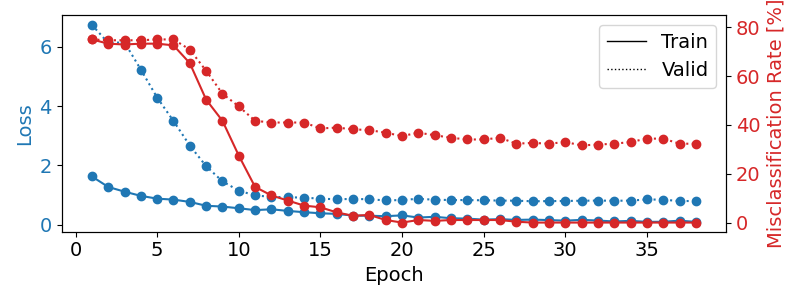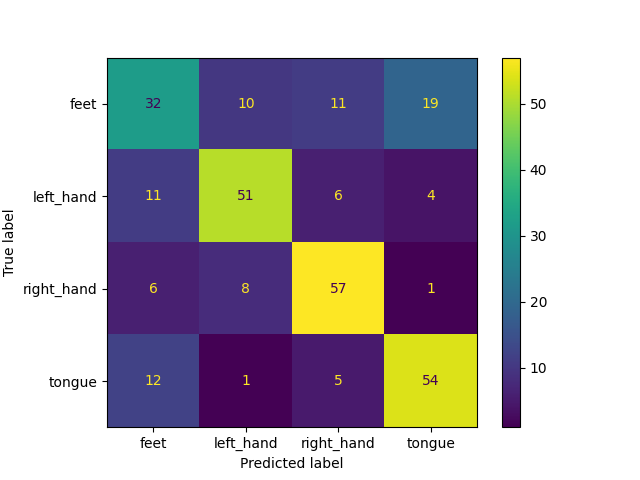# Trialwise Decoding on BCIC IV 2a Dataset#

This tutorial shows you how to train and test deep learning models with Braindecode in a classical EEG setting: you have trials of data with labels (e.g., Right Hand, Left Hand, etc.).

First, we load the data. In this tutorial, we use the functionality of braindecode to load datasets through MOABB to load the BCI Competition IV 2a data.

Note

To load your own datasets either via mne or from preprocessed X/y numpy arrays, see MNE Dataset Tutorial and Numpy Dataset Tutorial.

```from braindecode.datasets import MOABBDataset

subject_id = 3
dataset = MOABBDataset(dataset_name="BNCI2014001", subject_ids=[subject_id])
```

### Preprocessing#

Now we apply preprocessing like bandpass filtering to our dataset. You can either apply functions provided by mne.Raw or mne.Epochs or apply your own functions, either to the MNE object or the underlying numpy array.

Note

These prepocessings are now directly applied to the loaded data, and not on-the-fly applied as transformations in PyTorch-libraries like torchvision.

```from braindecode.preprocessing import (
exponential_moving_standardize, preprocess, Preprocessor)
from numpy import multiply

low_cut_hz = 4.  # low cut frequency for filtering
high_cut_hz = 38.  # high cut frequency for filtering
# Parameters for exponential moving standardization
factor_new = 1e-3
init_block_size = 1000
# Factor to convert from V to uV
factor = 1e6

preprocessors = [
Preprocessor('pick_types', eeg=True, meg=False, stim=False),  # Keep EEG sensors
Preprocessor(lambda data: multiply(data, factor)),  # Convert from V to uV
Preprocessor('filter', l_freq=low_cut_hz, h_freq=high_cut_hz),  # Bandpass filter
Preprocessor(exponential_moving_standardize,  # Exponential moving standardization
factor_new=factor_new, init_block_size=init_block_size)
]

# Transform the data
preprocess(dataset, preprocessors)
```
```/home/runner/work/braindecode/braindecode/braindecode/preprocessing/preprocess.py:55: UserWarning: Preprocessing choices with lambda functions cannot be saved.
warn('Preprocessing choices with lambda functions cannot be saved.')

<braindecode.datasets.moabb.MOABBDataset object at 0x7f50aaa1ed50>
```

### Cut Compute Windows#

Now we cut out compute windows, the inputs for the deep networks during training. In the case of trialwise decoding, we just have to decide if we want to cut out some part before and/or after the trial. For this dataset, in our work, it often was beneficial to also cut out 500 ms before the trial.

```from braindecode.preprocessing import create_windows_from_events

trial_start_offset_seconds = -0.5
# Extract sampling frequency, check that they are same in all datasets
sfreq = dataset.datasets.raw.info['sfreq']
assert all([ds.raw.info['sfreq'] == sfreq for ds in dataset.datasets])
# Calculate the trial start offset in samples.
trial_start_offset_samples = int(trial_start_offset_seconds * sfreq)

# Create windows using braindecode function for this. It needs parameters to define how
# trials should be used.
windows_dataset = create_windows_from_events(
dataset,
trial_start_offset_samples=trial_start_offset_samples,
trial_stop_offset_samples=0,
)
```

### Split dataset into train and valid#

We can easily split the dataset using additional info stored in the description attribute, in this case `session` column. We select `session_T` for training and `session_E` for validation.

```splitted = windows_dataset.split('session')
train_set = splitted['session_T']
valid_set = splitted['session_E']
```

## Create model#

Now we create the deep learning model! Braindecode comes with some predefined convolutional neural network architectures for raw time-domain EEG. Here, we use the shallow ConvNet model from Deep learning with convolutional neural networks for EEG decoding and visualization. These models are pure PyTorch deep learning models, therefore to use your own model, it just has to be a normal PyTorch nn.Module.

```import torch
from braindecode.util import set_random_seeds
from braindecode.models import ShallowFBCSPNet

cuda = torch.cuda.is_available()  # check if GPU is available, if True chooses to use it
device = 'cuda' if cuda else 'cpu'
if cuda:
torch.backends.cudnn.benchmark = True
# Set random seed to be able to roughly reproduce results
# Note that with cudnn benchmark set to True, GPU indeterminism
# may still make results substantially different between runs.
# To obtain more consistent results at the cost of increased computation time,
# you can set `cudnn_benchmark=False` in `set_random_seeds`
# or remove `torch.backends.cudnn.benchmark = True`
seed = 20200220
set_random_seeds(seed=seed, cuda=cuda)

n_classes = 4
# Extract number of chans and time steps from dataset
n_chans = train_set.shape
input_window_samples = train_set.shape

model = ShallowFBCSPNet(
n_chans,
n_classes,
input_window_samples=input_window_samples,
final_conv_length='auto',
)

# Send model to GPU
if cuda:
model.cuda()
```

## Training#

Now we train the network! EEGClassifier is a Braindecode object responsible for managing the training of neural networks. It inherits from skorch.NeuralNetClassifier, so the training logic is the same as in Skorch.

Note: In this tutorial, we use some default parameters that we have found to work well for motor decoding, however we strongly encourage you to perform your own hyperparameter optimization using cross validation on your training data.

```from skorch.callbacks import LRScheduler
from skorch.helper import predefined_split

from braindecode import EEGClassifier
# These values we found good for shallow network:
lr = 0.0625 * 0.01
weight_decay = 0

# For deep4 they should be:
# lr = 1 * 0.01
# weight_decay = 0.5 * 0.001

batch_size = 64
n_epochs = 4

clf = EEGClassifier(
model,
criterion=torch.nn.NLLLoss,
train_split=predefined_split(valid_set),  # using valid_set for validation
optimizer__lr=lr,
optimizer__weight_decay=weight_decay,
batch_size=batch_size,
callbacks=[
"accuracy", ("lr_scheduler", LRScheduler('CosineAnnealingLR', T_max=n_epochs - 1)),
],
device=device,
)
# Model training for a specified number of epochs. `y` is None as it is already supplied
# in the dataset.
clf.fit(train_set, y=None, epochs=n_epochs)
```
```  epoch    train_accuracy    train_loss    valid_accuracy    valid_loss      lr     dur
-------  ----------------  ------------  ----------------  ------------  ------  ------
1            0.2535        1.6382            0.2500        5.2101  0.0006  6.2506
2            0.2708        1.2828            0.2465        5.6990  0.0005  6.1431
3            0.2604        1.1267            0.2500        5.0720  0.0002  6.1103
4            0.2708        1.1386            0.2500        4.2096  0.0000  6.2784

<class 'braindecode.classifier.EEGClassifier'>[initialized](
module_=ShallowFBCSPNet(
(ensuredims): Ensure4d()
(dimshuffle): Expression(expression=transpose_time_to_spat)
(conv_time): Conv2d(1, 40, kernel_size=(25, 1), stride=(1, 1))
(conv_spat): Conv2d(40, 40, kernel_size=(1, 22), stride=(1, 1), bias=False)
(bnorm): BatchNorm2d(40, eps=1e-05, momentum=0.1, affine=True, track_running_stats=True)
(conv_nonlin_exp): Expression(expression=square)
(pool): AvgPool2d(kernel_size=(75, 1), stride=(15, 1), padding=0)
(pool_nonlin_exp): Expression(expression=safe_log)
(drop): Dropout(p=0.5, inplace=False)
(conv_classifier): Conv2d(40, 4, kernel_size=(69, 1), stride=(1, 1))
(softmax): LogSoftmax(dim=1)
(squeeze): Expression(expression=squeeze_final_output)
),
)
```

## Plot Results#

Now we use the history stored by Skorch throughout training to plot accuracy and loss curves.

```import matplotlib.pyplot as plt
from matplotlib.lines import Line2D
import pandas as pd

# Extract loss and accuracy values for plotting from history object
results_columns = ['train_loss', 'valid_loss', 'train_accuracy', 'valid_accuracy']
df = pd.DataFrame(clf.history[:, results_columns], columns=results_columns,
index=clf.history[:, 'epoch'])

# get percent of misclass for better visual comparison to loss
df = df.assign(train_misclass=100 - 100 * df.train_accuracy,
valid_misclass=100 - 100 * df.valid_accuracy)

plt.style.use('seaborn')
fig, ax1 = plt.subplots(figsize=(8, 3))
df.loc[:, ['train_loss', 'valid_loss']].plot(
ax=ax1, style=['-', ':'], marker='o', color='tab:blue', legend=False, fontsize=14)

ax1.tick_params(axis='y', labelcolor='tab:blue', labelsize=14)
ax1.set_ylabel("Loss", color='tab:blue', fontsize=14)

ax2 = ax1.twinx()  # instantiate a second axes that shares the same x-axis

df.loc[:, ['train_misclass', 'valid_misclass']].plot(
ax=ax2, style=['-', ':'], marker='o', color='tab:red', legend=False)
ax2.tick_params(axis='y', labelcolor='tab:red', labelsize=14)
ax2.set_ylabel("Misclassification Rate [%]", color='tab:red', fontsize=14)
ax2.set_ylim(ax2.get_ylim(), 85)  # make some room for legend
ax1.set_xlabel("Epoch", fontsize=14)

# where some data has already been plotted to ax
handles = []
handles.append(Line2D(, , color='black', linewidth=1, linestyle='-', label='Train'))
handles.append(Line2D(, , color='black', linewidth=1, linestyle=':', label='Valid'))
plt.legend(handles, [h.get_label() for h in handles], fontsize=14)
plt.tight_layout()
```## Plot Confusion Matrix#

Generate a confusion matrix as in https://onlinelibrary.wiley.com/doi/full/10.1002/hbm.23730

```from sklearn.metrics import confusion_matrix
from braindecode.visualization import plot_confusion_matrix

# generate confusion matrices
# get the targets
y_pred = clf.predict(valid_set)

# generating confusion matrix
confusion_mat = confusion_matrix(y_true, y_pred)

# label_dict is class_name : str -> i_class : int
label_dict = valid_set.datasets.windows.event_id.items()
# sort the labels by values (values are integer class labels)
labels = list(dict(sorted(list(label_dict), key=lambda kv: kv)).keys())

# plot the basic conf. matrix
plot_confusion_matrix(confusion_mat, class_names=labels)
``````<Figure size 800x550 with 1 Axes>
```

Total running time of the script: ( 0 minutes 41.558 seconds)

Estimated memory usage: 772 MB

Gallery generated by Sphinx-Gallery